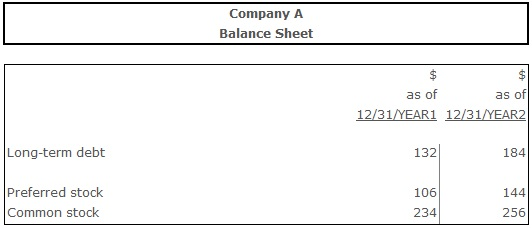# Long-Term Debt to Total Capitalization Ratio

Long-Term Debt to Total Capitalization Ratio - a ratio measuring the financial leverage of a firm by dividing the long-term debt by the amount of capital available.

## Formula(s):

Long-Term Debt to Total Capitalization Ratio = Long-Term Debt ÷ (Long-Term Debt + Preferred Equity + Common Equity)

## Example:Long-Term Debt to Total Capitalization Ratio (Year 1) = 132 ÷ (132 + 106 + 234) = 0,28

Long-Term Debt to Total Capitalization Ratio (Year 2) = 184 ÷ (184 + 144 + 256) = 0,31

## Conclusion:

Year 2 witnessed the increase of the long-term debt to total capitalization ratio from 0,28 to 0,31 comparing to year 1. This means that the company has become more dependent on the debt in terms of finance.